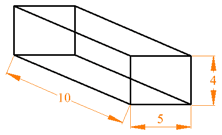Page

# Volume

Volume is the measure of the inside of a 3-D figure.

The amount of 3-dimensional space an object occupies. Capacity.For this example the volume is 200 units3

The formula is V=Bh
B=Area of the Base (use the STAAR Chart to find B)
h=height of Prism

Units of volume include:
Metric: cubic centimeters (cm3), cubic meters (m3)

Practice Volume here and here .

# Surface AreaThe total area of the surface of a three-dimensional object.

Example: the surface area of a cube is the area of all 6 faces added together.
The formula is S = Ph + 2B

P=Perimeter of the Base
h=height of Prism
B=Area of the Base (use the STAAR Chart to find B)
Units of volume include:
Metric: cubic centimeters (cm2), cubic meters (m2),

Practice Surface Area here.